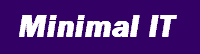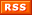Research, training, consultancy and software to reduce IT costs
 Home Site map Newsletter index Integration System governance
 Previous | Next Printer friendly
23 January 2007

## Justifying IT investment 3: the value of compliance

To calculate a financial benefit for any IT investment, we have to put a value on meeting our own objectives.

Last week I outlined how to estimate the financial benefit of purely IT investments, or IT infrastructure. This week I show how to estimate the unit cost and difficulty figures that we need to plug into the calculations. (You might like to read last week's newsletter as an introduction to this.)

The general approach is to estimate the difficulty of IT, and to use this to calculate unit cost.

To estimate the difficulty of our IT, ask the question, "If all our systems met our objectives perfectly, how much cheaper would they be?" This requires some educated guesswork. It is worth rephrasing the question in many ways, such as the questions below.

• If systems were perfectly structured and documented, how much less would we spend on maintenance?
• If data was not duplicated unnecessarily, how much would we save on disk space and backup?
• If systems ran as efficiently as they could, how much less CPU and memory would they need?
• If systems were resilient, intuitive, and never gave misleading errors, how much would our help desk costs be reduced?

Of course it depends on your situation. Try a poll of your IT managers to come to a consensus.

Convert your estimate of the value of meeting your objectives into the "base difficulty" figure we need by using the formula I introduced last week. This states that cost is proportional to difficulty, and that difficulty can be calculated as:

difficulty = base difficulty + ( 100 - score )

Base difficulty is a measure of the difficulty of running your IT under perfect conditions. If you have an overall system governance score for your systems, and an estimate of how much your IT would cost under perfect conditions (when score is 100), you can calculate base difficulty.

For example, if you think that under perfect conditions IT would cost 60% of the current cost, and your overall score is 70%, your can calculate your base difficulty by solving the equations.

current difficulty = base difficulty + ( 100 - 70 )

current difficulty * 0.6 = base difficulty

Which after a little algebra resolves to.

base difficulty = 45

Use the base difficulty to calculate unit cost. The overall cost equation is:

cost = volume x ( base difficulty + ( 100 - score )) x unit cost

If you have an annual IT cost of £12,000,000, a volume of 250, a score of 75, and a base difficulty of 25, your calculation would be:

12,000,000 = 250 x ( 25 + ( 100 - 75 ) ) x unit cost

Which gives a unit cost of £960.

Now, at last, we have the unit cost figure to plug into last weeks equation.

benefit = volume x improvement in score x unit cost

For example, if volume of IT is 250 and unit cost is £960, an IT investment that increases the score from 75.0 to 76.2 would be valued at:

benefit = 250 x ( 76.2 - 75.0 ) x £960 = £288,000 per year

We now have everything we need to make a financial benefit case for purely IT investment. Next week I will summarise what we have covered, and the benefits of this method of analysis.

Next: Justifying IT investment 4: summary

### Subscription

To subscribe to the newlsetter, simply send an email to newsletter-subscribe@minimalit.com.